# 產品經理：你能不能用div給我畫條龍？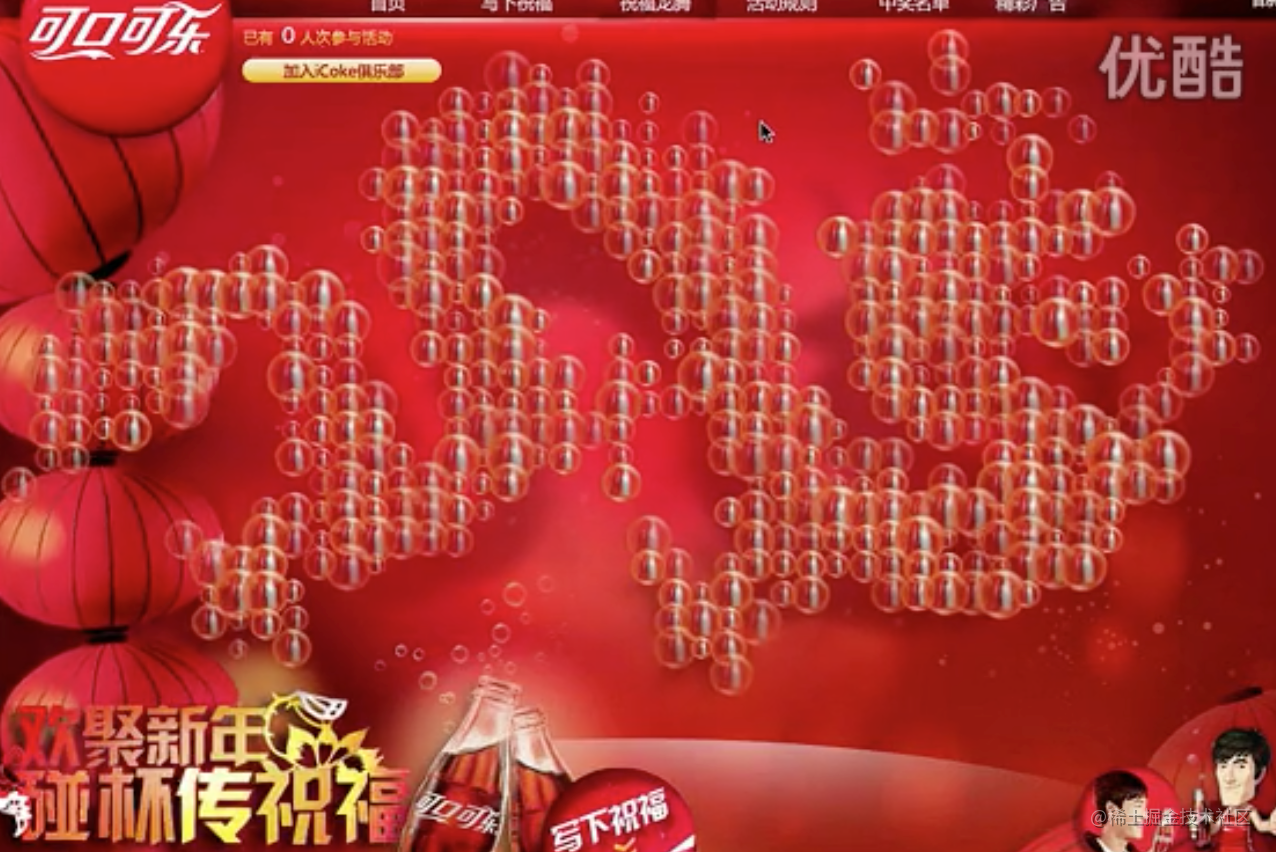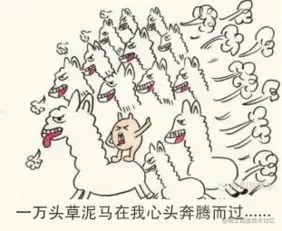# 龍從哪裡來？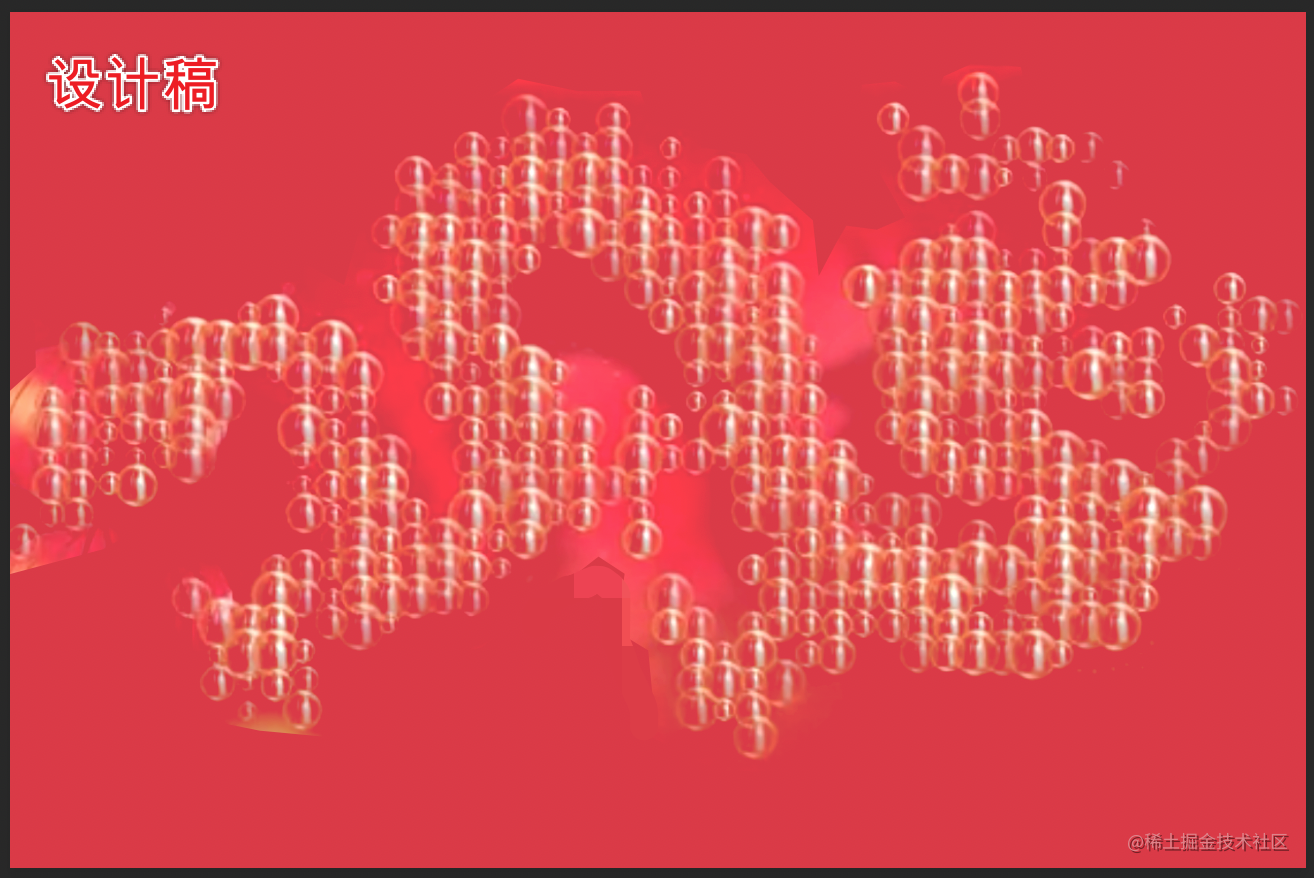# 拆解需求

• 需求1：有滑鼠互動效果（太簡單）
• 需求2：氣泡要浮動（css動畫，easy）
• 需求3：氣泡組成一條龍

`誒，畫個龍`

`用什麼畫，canvas`

`canvas能獲得指定區域的畫素點陣`

# 程式碼時間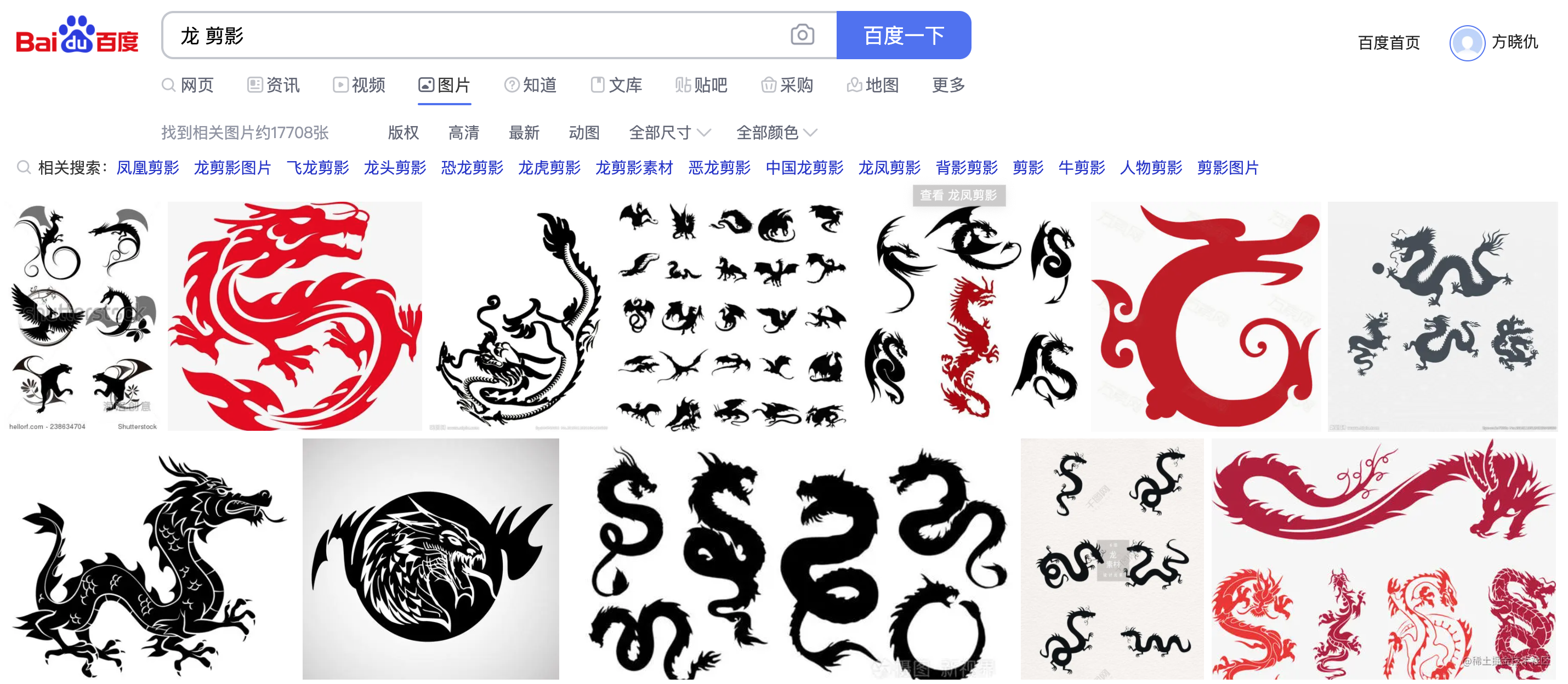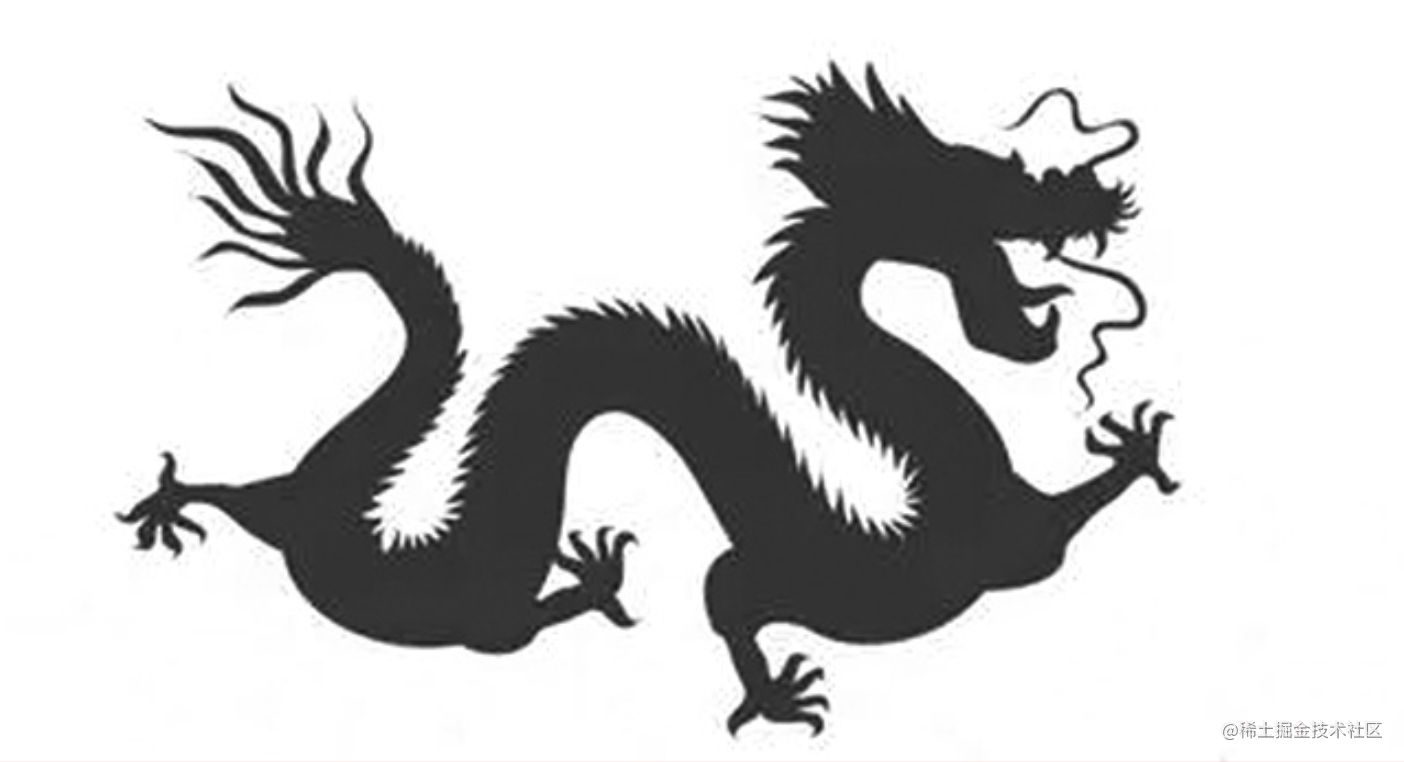## 將圖片繪製到canvas中

~~~javascript var canvas = document.getElementById("canvas"); var ctx = canvas.getContext("2d");

var image = new Image(); image.src = "dragon.jpg"; image.onload = function(){ canvas.width = image.width; canvas.height = image.height;

``````    ctx.drawImage(image,0,0);
``````

} ~~~

## 獲取並裁剪畫布的點陣資訊

~~~javascript var imageData = ctx.getImageData(0,0,image.width,image.height).data; ctx.fillStyle = "#ffffff"; ctx.fillRect(0,0,image.width,image.height);

var gap = 6;

for (var h = 0; h < image.height; h+=gap) { for(var w = 0; w < image.width; w+=gap){ var position = (image.width * h + w) * 4; var r = imageData[position], g = imageData[position + 1], b = imageData[position + 2];

``````        if(r+g+b==0){
ctx.fillStyle = "#000";
ctx.fillRect(w,h,4,4);
}
}
``````

} ~~~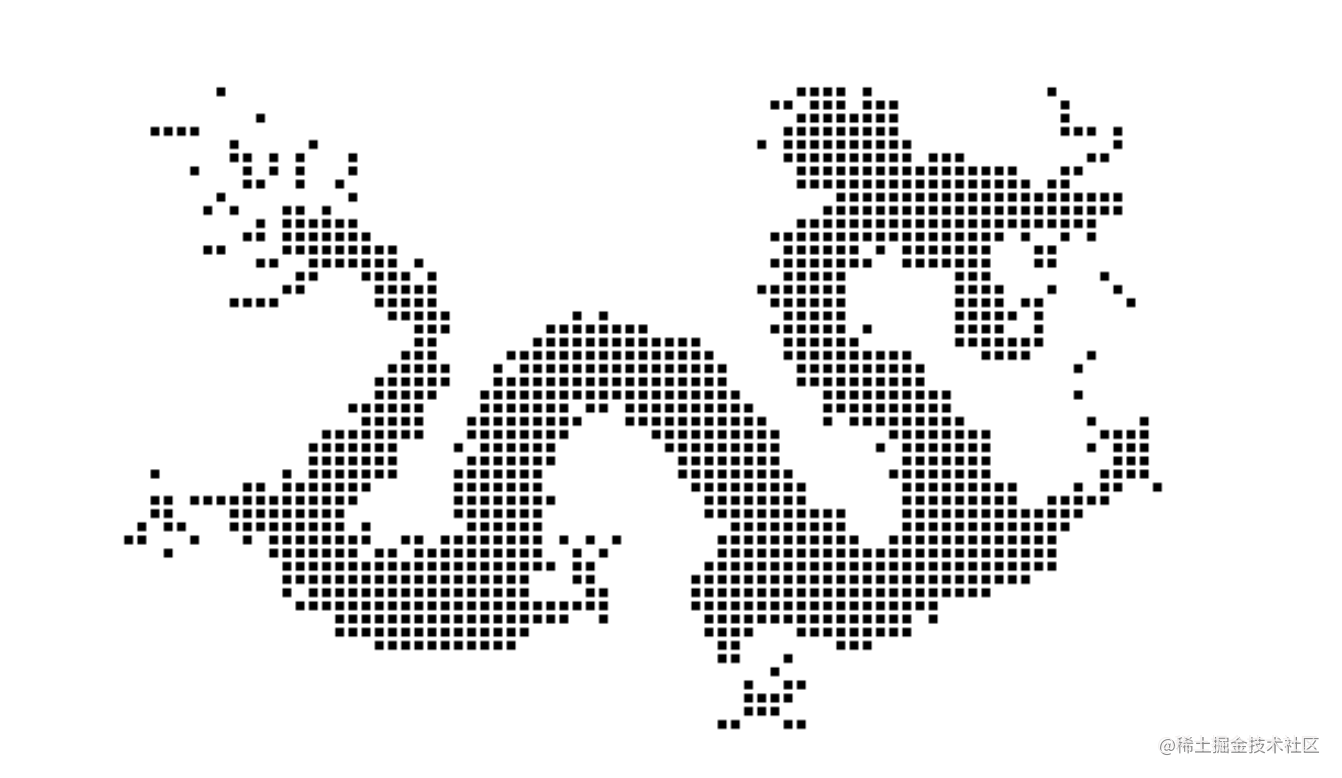## 通過點陣資訊生成氣泡dom

~~~javascript var dragonContainer = document.getElementById("container"); var dragonScale = 2;

for (var h = 0; h < image.height; h+=gap) { for(var w = 0; w < image.width; w+=gap){ var position = (image.width * h + w) * 4; var r = imageData[position], g = imageData[position + 1], b = imageData[position + 2];

``````        if(r+g+b==0){
var bubble = document.createElement("img");
bubble.src = "bubble.png";
bubble.setAttribute("class","bubble");

var bubbleSize = Math.random()*10+20;
bubble.style.left = (w*dragonScale-bubbleSize/2) + "px";
bubble.style.top = (h*dragonScale-bubbleSize/2) + "px";
bubble.style.width = bubble.style.height = bubbleSize+"px";
bubble.style.animationDuration = Math.random()*6+4 + "s";

dragonContainer.appendChild(bubble);
}
}
``````

} ~~~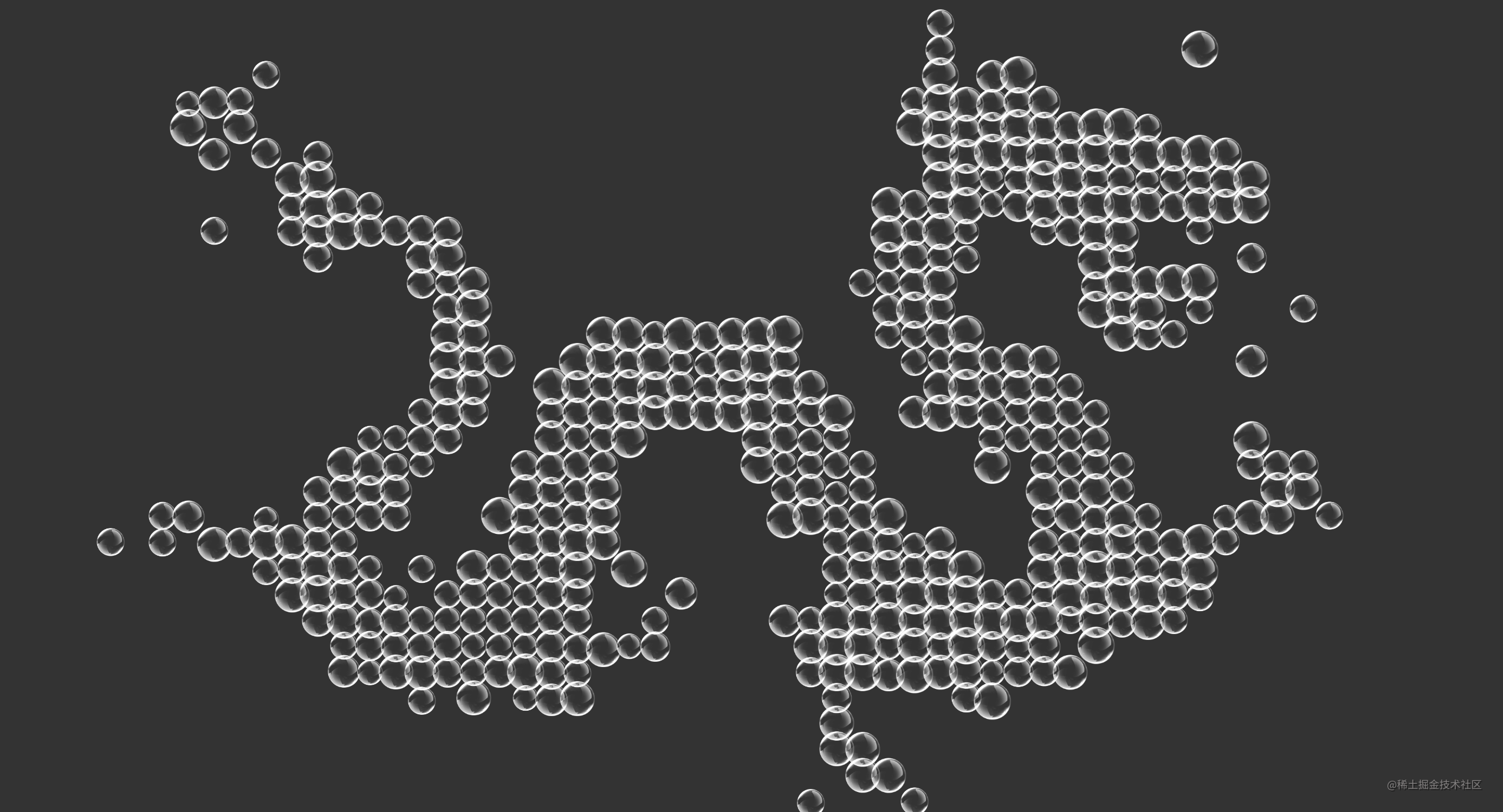# 開心摸魚吧

• 嗶哩嗶哩：`大帥老猿`
• 微信公眾號：`大帥老猿`